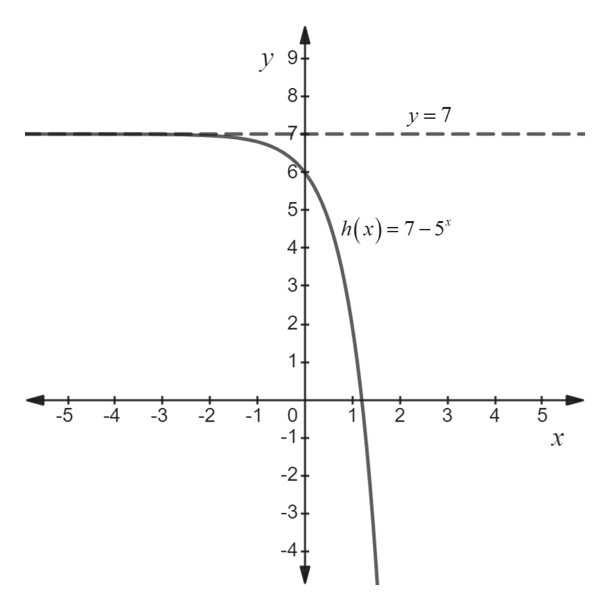# Graph the function. Find the domain, range, and asymptoteh(x) = 7 − 5x

Question
3 views

Graph the function. Find the domain, range, and asymptote

h(x) = 7 − 5x

check_circle

Step 1

The domain of a function is the set of all real values of x that will give real values for y.

The range of a function is the set of all real values of y that can get by plugging real numbers into ...help_outlineImage Transcriptionclosey 9f 8+ y=7 6 5+ h(x)=7-5* 4+ 3+ 24 1+ -5 -4 2 -1 -1 1 2 3 4 x -2+ -3+ -4 +LO LO fullscreen

### Want to see the full answer?

See Solution

#### Want to see this answer and more?

Solutions are written by subject experts who are available 24/7. Questions are typically answered within 1 hour.*

See Solution
*Response times may vary by subject and question.
Tagged in

### Other